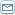Fibonacci Forex Trading - An Introduction

 By: Dave HikadeOne of the most intriguing, if not mysterious, aspects of Forex Trading is the application of Fibonacci numbers in technical anaysis of the market.If you have heard the term "Fibonacci" but are not very familiar with it or how it relates to trading, then sit back, and read this series of two articles which will help explain this mathematicalrelationship to you.To begin with, Fibonacci is actually the name of a well known mathematician who lived from 1175 to 1250 A.D. He made great contributions to the world and introduced the decimal system to Europe.The so called Fibonacci number sequence first appeared as the solution to a problem in the Liber Abaci, which was a book written by the mathematician in 1202 and introduced the Arabic numerals presently used to Europe when it was limited to Roman numerals.The actual problem that was solved with this famous numerical series dealt with the propagation of rabbits, of all things. The question to be solved was essentially, starting with only one pair of rabbits, how many pair could be generated if each mature pair "delivers" a new pair each month, which itself becomes productive in the second month.The solution starts with a 0 and 1, and each new number is the sum of the previous two numbers(0 + 1 = 1; 1 + 0 = 1; 1 + 1 = 2; 1 + 2 = 3; 3 + 2 = 5; etc). Or as a more acceptable expressionin the world of mathematics: Fn+1 = Fn + Fn-1.This leads to the following infinite series: 0, 1, 1, 2, 3, 5, 8, 13, 21, 34, 55, 89, 144, etc.Fibonacci found that this series of numbers and their ratios to each other surprisingly are prevalent throughout nature and can be found even in human nature.The ratio of any number to the next larger number in this series (e.g., 55 to 89), approaches 0.618, or 61.8 %, and this as well as its inverse (0.382 or 38.2 %) become important Fibonacci retracement numbers which will be further discussed in the next article.Likewise, the ratio of the next larger number to any number in the series (e.g., 89 to 55) approaches 1.618, and this very significant number is known as the "golden ratio", "golden mean", and "divine proportion", among other names.This number was highly significant to both Greek and Egyptian cultures and had important implications in areas of art and science. It was also utilised in the construction of many buildings in those cultures, including the pyramids and the parthenon. This ratio can even be found in the Holy Bible. Two such examples are the ratios in the proportions of the Ark of the Covenant (Exodus 25:10) and the construction of Noah's Ark (Genesis 6:15).This ratio (1.618), also known as "phi", is found all around the world today.For instance, in the human body, the proportion of the distance from the head to the finger tips vs the total body height has this ratio. Likewise, the distance from the navel to the elbows vs. the distance from the head to the finger tips.Even the program for all life on this planet, the DNA molecule, is based on this same ratio. It measures34 Angstroms (a very small unit of measurement) long by 21 Angstroms wide for each full cycle in the double helix spiral. As can be seen in the series shown above, 34 and 21 are successive Fibonacci numbers with their ratio approaching phi.One final, galactic example is in recent (2003)studies based on data taken from NASA's Wilkinson Microwave Anisotropy Probe (WMAP) on cosmic radiation background that suggest that the universe is finite and shaped like a dodecahedron. This geometric shape is based on pentagons, which are based on phi.Many more examples could be given which demonstrate how the world and our lives are impacted by the applications of Fibonacci numbers but I think you get the point in the few examples that were given here.The next logical question becomes: what does this interesting numerical series have to do with Forex Trading, especially for YOU.